# # Location Analysis

## # 1 Julia Set

Original question on math.stackexchange.com: How can I reconstruct a Julia set by a given image.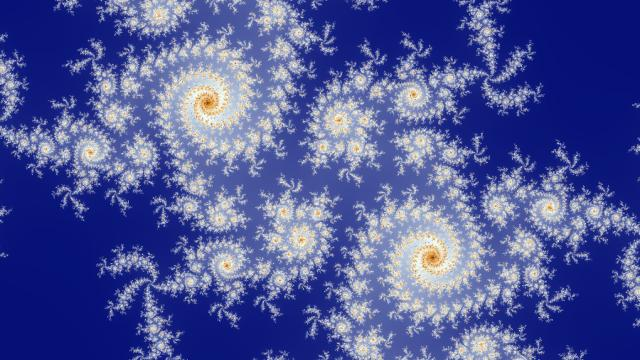The image may be a zoom into a quadratic Julia set for $$f_c(z) = z^2 + c$$ near $$c = 0.270723273 + 0.575139611 i$$, centered on $$0$$ with zoom factor $$5.3$$ (zoom factor $$1$$ would have $$\pm i$$ at the top and bottom edges of the image). I found this by browsing in the Mandelbrot set.

The “birds feet” have $$4$$ spokes, so I looked around the period $$4$$ bulb attached to the main cardioid at the top right.

Then, the central spirals have $$3$$ arms so I looked around its child period $$12$$ bulb at internal angle $$\frac{2}{3}$$ (anticlockwise from the antenna of the period $$4$$ bulb).

Then, these spirals are quite curly, so I looked near the base of the component where it attaches to its parent. I looked on the anticlockwise side and got lucky in the direction of the turn of the spirals, they would spiral in the other direction on the other side of the bond point.

Because the Julia set is disconnected, the $$c$$ value is outside the Mandelbrot set, and I zoomed in until the image matched: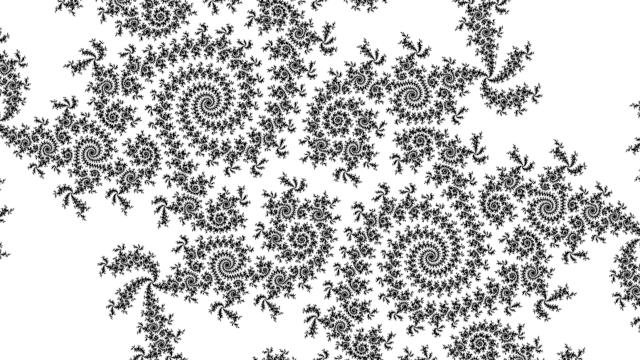## # 2 Embedded Julia Set

Original question on math.stackexchange.com: How can I reconstruct a Julia set by a given image.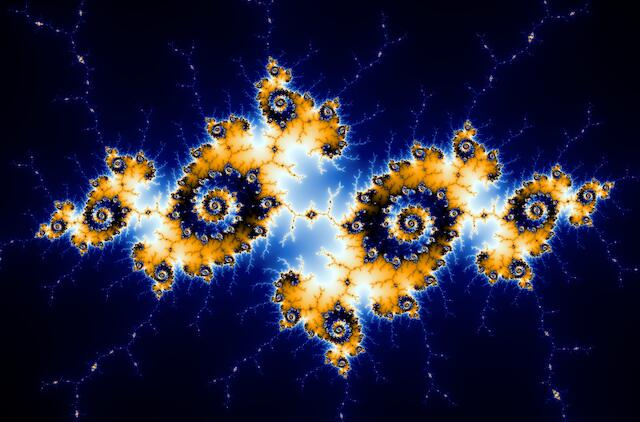The image is likely an embedded Julia set in the Mandelbrot set, because of the decorations (and filename: Mandelbrot_set_image.png).

The decorations have a 3-way branching structure, so look near the 1/3 bulb (it turns out that the 2/3 bulb’s branches are oppositely oriented).

Embedded Julia sets occur when zooming near a miniature Mandelbrot set copy. The filament structure seems nothing special, so pick the largest, the period 4 one in the longest filament. We will look for embedded Julia sets in the hairs decorating it.

Now look at the spirals. 13 steps around the spiral is the same as 2 steps in the radial direction, so look for a q/13 child bulb near the 1/2 bulb. I went first for q=6 which seemed ok, the ends of the spirals in nearby embedded Julia sets also have 5 and 6 features on them before the main filament connecting to the center or next spiral out.

The 6/13 bulb has 13 spokes, not knowing any better pick the one with the longest hair. Looking at the center of the image, there are two filaments branching in the opposite direction to the rest. This indicates that we should zoom into the sideways sticky outtie bit some way down the filament. Counting branches tells how far.

Looking at the very center of the image, it appears with 2-fold symmetry, not 4-fold as topmost embedded Julia sets have. Here we cannot see clearly enough due to the image resolution, but similar shapes can be found by zooming towards a spiral center, towards a node in the filaments and then off center to an embedded Julia set form in its hairs.

Putting all of that into practice gives $c_0 \approx -0.16016174539934025+1.0375719438634177i$ though many other places nearby will look similar.

You can explore interactively at mandelbrot/web (No way to zoom out apart from editing the view radius by hand.)

Here is a screenshot: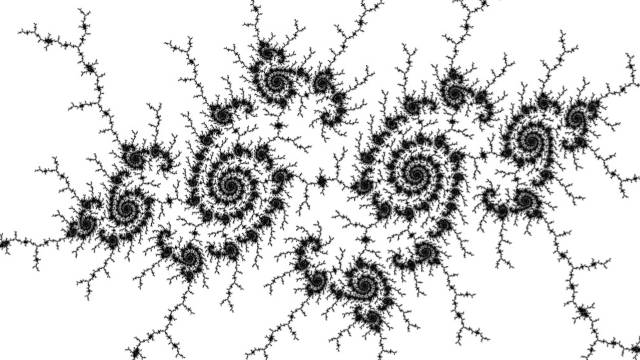## # 3 Mercator Mandelbrot

Original thread on fractalforums.org: Can you find the location of this fractal?.

Original image under CC-BY license on flickr.com: “Mercator Mandelbrot” by Anders Sandberg: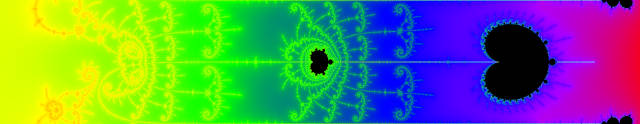The original image mirrored on Wikimedia Commons has dimensions $$3101 \times 601$$ pixels.

The location is to be exterior to the Mandelbrot set but close to the real axis, because the real axis antenna extends along the edges from the top level continent on the right almost all the way to the left, and the left edge of image has no boundary.

The period $$2$$ circle centered on $$-1$$ with radius $$1/4$$ takes up $$66$$ of $$601$$ vertical pixels representing $$1$$ turn. By trigonometry, $c \approx -1 - 0.25 / \sin\left(\pi \frac{66}{601}\right) \approx -1.739 \pm 0.005.$

This is close to the cusp of the period $$3$$ island at $$-1.75$$. Its period $$6$$ bulb has center $$-1.7728929033816239$$ and diameter $$8.6922294830907298\times 10^{-3}$$. In the picture the bulb measures $$35$$ of $$601$$ vertical pixels. By trigonometry, $c \approx -1.7728929033816239 + 8.6922294830907298\times10^{-3}/2 / \sin\left(\pi\frac{35}{601}\right) \approx -1.7490 \pm 0.00035.$

Looking at the spirals surrounding the next minibrot, it has $$14$$ hairs in one full turn.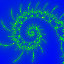Looking for similar geometry in the features surrounding minis on the cusp hair of the period $$3$$ mini I found period $$38$$ at $$-1.748764576304968589755294$$: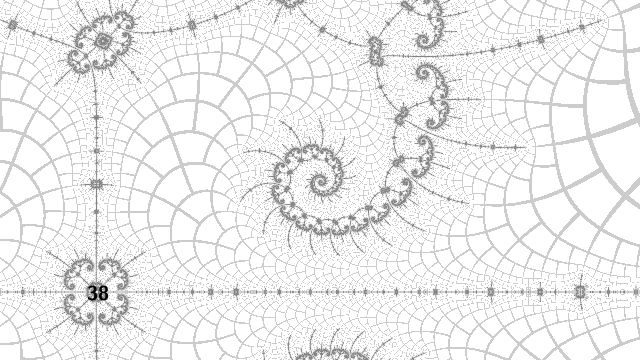This might be the third minibrot from the right in the original image. The zoomed point is near the hair from its cusp, between $$16$$x and $$32$$x feature doubling levels, just off the axis.

Guessing a bit: there is a period $$189$$ mini at $$-1.748764520196145650648490036653848$$ with atom domain size $$1.3 \times 10^{-12}$$, which may be near the center of the original image.

Zooming between the $$8$$x and $$16$$x doubling levels of features surrounding this minibrot, into empty space just off the real axis: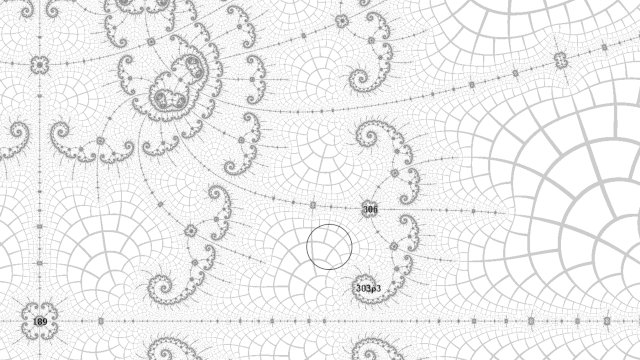This is roughly the right zoom depth, near the limit of double precision…

Final recreated image with $c = -1.748764520194788535 + 3.039776013681213845 \times 10^{-13} i.$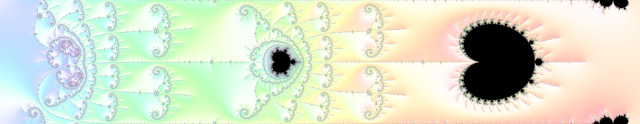Not perfect at the left hand edge, and doesn’t fully match up perfectly with the original image when overlayed.

### # 3.1 Parameter Files

#### # 3.1.1 m-perturbator-gtk

program = "m-perturbator-gtk"
version ="v0.2-3-g57bae2f"
width = 1705
height = 1128
theme = "light"
colour = "low"
key = "none"
view.default = { precision = 57, re = "-1.748764520194788535e+00", im = "3.039776013681213845e-13", radius = "3.4165983035677679e-13", iterations = 65536 }
annotations = [
{ style = { rgb = [ 0.000000, 0.000000, 0.000000 ], fill_type = -1, line_type = 0 }, label = "1", type = "nucleus", precision = 53, re = "0.0000000000000000e+00", im = "0.0000000000000000e+00", period = 1, domain_size = "0.0000000000000000e+00", size = "1.0000000000000000e+00" },
{ style = { rgb = [ 0.000000, 0.000000, 0.000000 ], fill_type = -1, line_type = 0 }, label = "2", type = "nucleus", precision = 53, re = "-1.0000000000000000e+00", im = "0.0000000000000000e+00", period = 2, domain_size = "1.0000000000000000e+00", size = "5.0000000000000000e-01" },
{ style = { rgb = [ 0.000000, 0.000000, 0.000000 ], fill_type = -1, line_type = 0 }, label = "6", type = "nucleus", precision = 59, re = "-1.772892903381623801e+00", im = "4.154310888769911952e-66", period = 6, domain_size = "1.738071450241106735e-02", size = "8.6922294830908287e-03" },
{ style = { rgb = [ 0.000000, 0.000000, 0.000000 ], fill_type = -1, line_type = 0 }, label = "3", type = "nucleus", precision = 58, re = "-1.754877666246692759e+00", im = "0.000000000000000000e+00", period = 3, domain_size = "2.344867659879372908e-01", size = "1.9035515913132451e-02" },
{ style = { rgb = [ 0.000000, 0.000000, 0.000000 ], fill_type = -1, line_type = 0 }, label = "38", type = "nucleus", precision = 77, re = "-1.748764576304968589755294e+00", im = "-2.220285011212775056653662e-94", period = 38, domain_size = "2.782764159659592060377583e-06", size = "5.0696314983625103e-08" },
{ style = { rgb = [ 0.000000, 0.000000, 0.000000 ], fill_type = -1, line_type = 0 }, label = "189", type = "nucleus", precision = 107, re = "-1.748764520196145650648490036653848e+00", im = "1.492455316855394121268545849147436e-112", period = 189, domain_size = "1.295935816452428383650945447912832e-12", size = "4.2036885942657974e-17" },
{ style = { rgb = [ 0.000000, 0.000000, 0.000000 ], fill_type = -1, line_type = 0 }, label = "306", type = "nucleus", precision = 124, re = "-1.74876452019455478905715444315469562960e+00", im = "5.39055655826750907950005827944318155180e-13", period = 306, domain_size = "3.32163855383685952253255672299332623102e-15", size = "2.7614778398698583e-22" },
{ style = { rgb = [ 0.000000, 0.000000, 0.000000 ], fill_type = -1, line_type = 0 }, label = "303p3", type = "misiurewicz", precision = 60, re = "-1.7487645201945641611e+00", im = "1.6236001555905457669e-13", preperiod = 303, period = 3, domain_size = "8.6174683690852703e-15" },
]


#### # 3.1.2 fraktaler-3

program = "fraktaler-3"
version = "0-414-g61fa84a+"
location.real = "-1.748764520194788535"
location.imag = "3e-13"
location.zoom = "1e15"
bailout.iterations = 262144
bailout.maximum_reference_iterations = 262144
bailout.maximum_perturb_iterations = 4096
image.width = 601
image.height = 67
image.subframes = 64
opencl.tile_width = 601
opencl.tile_height = 67
transform.reflect = true
transform.exponential_map = true
render.zoom_out_sequence = true


#### # 3.1.3 Post-Processing Script

fraktaler-3 --batch --no-persistence mercator-mandelbrot.f3.toml
for i in *.exr ; do convert $i -colorspace sRGB$i.ppm ; done
pnmcat -tb *.exr.ppm > mercator-mandelbrot.ppm
gimp mercator-mandelbrot.ppm
# rotate, crop, export as 8-bit PNG